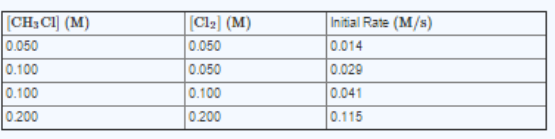# Problem: The data below were collected for the following reaction: CH3Cl(g) + 3 Cl2(g) → CCl4(g) + 3 HCl(g)Part A Write an expression for the reaction rate Law. Part B CaIculate vaIue of rate constant k. Express your answer using two significant figures.Part C What is overall order of the reaction? Express your answer using two significant figures.

###### FREE Expert Solution

We’re being asked to calculate the rate law and the value of the rate constant (k) based on the reaction and data given.

Recall that the rate law only focuses on the reactant concentrations and has a general form of:

k = rate constant
A & B = reactants
x & y = reactant orders

98% (212 ratings)###### Problem Details

The data below were collected for the following reaction:

CH3Cl(g) + 3 Cl2(g) → CCl4(g) + 3 HCl(g)Part A Write an expression for the reaction rate Law.

Part B CaIculate vaIue of rate constant k. Express your answer using two significant figures.

Part C What is overall order of the reaction? Express your answer using two significant figures.